# NCERT Solutions for Class 10 Science Chapter 5 - Electricity

##### Question 1:

What does an electric current mean?

Electric current is the electric charge flowing through a conductor in a unit time. Electric current,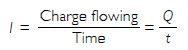##### Question 2:

Define the unit of current.

The SI unit of current is ampere. Current flowing through a conductor is said to be 1 ampere if 1 coulomb of charge flows through the conductor in 1 second.

##### Question 3:

Calculate the number of electrons constituting 1 coulomb of charge.

Charge on an electron = 1.6 × 10–19 C
1.6 × 10–19 C of charge is there on 1 electron,
Therefore, 1 C of charge is on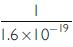##### Question 4:

Name a device that helps to maintain a potential difference across a conductor.

Electric cell is a device used to maintain a potential difference across the ends of a conductor.

##### Question 5:

What is meant by saying that the potential difference between two points is 1 V?

Potential difference between two points in a current carrying conductor is 1 volt if 1 joule of work is done to move a charge of 1 coulomb from one point to the other.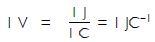##### Question 6:

How much energy is given to each coulomb of charge passing through a 6 V battery?

W = VQ = 6 volt × 1 coulomb =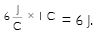##### Question 7:

How much energy is given to each coulomb of charge passing through a 15 V battery?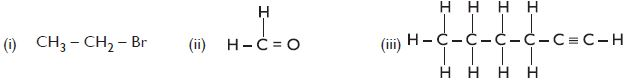W = VQ = 6 volt × 1 coulomb =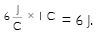##### Question 8:

On what factors does the resistance of a conductor depend?

Resistance R of a conductor depends upon length l, area A and nature of the conductor. It has been experimentally confirmed that: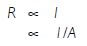Combining above equations, we have:

or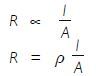where, r is a constant called specific resistance or electric resistivity of the material of the conductor.

##### Question 9:

Will the current flow more easily through a thick wire or a thin wire of the same material when connected to the same source? Why?

The resistance of a wire is inversely proportional to the area of cross-section. The thicker the wire, more is the area of cross section and lesser will be the resistance. Thus, current will flow more easily through a thicker wire of less resistance than through a thinner wire of more resistance.

##### Question 10:

Let the resistance of an electrical component remain constant while the potential difference across the two ends of the component decreases to half the former value. What change will occur in the current through it?

According to Ohm’s law, if the physical conditions remain constant, the current is directly proportional to the potential difference. Therefore, when potential difference is halved, the current will be halved as the resistance remains constant.

##### Question 11:

Why are coils of electric toasters and electric irons made of an alloy rather than that of a pure metal?

Resistivity (specific resistance) of alloys is much higher than that of pure metals. Alloys do not oxidise readily at very high temperatures. The alloys are, therefore, commonly used in electric heating devices like electric iron, electric toasters etc.

##### Question 12:

(a) Which among iron and mercury is a better conductor?
(b) Which material is the best conductor?

(a) Resistivity of iron is 10 × 10–8 ohm m and that of mercury is 94 × 10–8 ohm m, therefore, iron is a better conductor than mercury.
(b) Silver of resistivity 1.60 × 10–8 ohm m is the best conductor followed by copper with resistivity 1.62 × 10–8 ohm m.

##### Question 13:

Draw a schematic diagram of a circuit consisting of a battery of three cells of 2 V each, a 5 $\mathrm{\Omega }$ resistor; an 8 $\mathrm{\Omega }$ resistor and 12 $\mathrm{\Omega }$ resistor and a plug key, all connected in series.

The required diagram is as shown.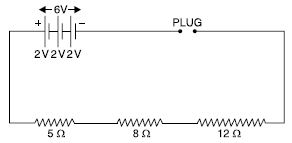##### Question 14:

Redraw the circuit given below, putting an ammeter to measure the current through the resistors and a voltmeter to measure the potential difference across the 12 W resistor. What would be the reading in the ammeter and the voltmeter?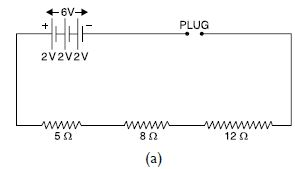Modified circuit is as shown in the figure (b). Since 5 W, 8 W and 12 W are in series, therefore the total resistance in series
Rs = R1 + R2 + R3 = 5 + 8 + 12 = 25 W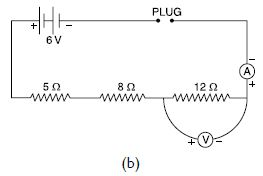Current through circuit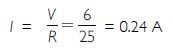\ Reading of ammeter = 0.24 A
P.D. across 12 W i.e., reading of voltmeter
= I.R
= 0.24 × 12
= 2.88 V

##### Question 15:

Judge the equivalent resistance when the following are connected in parallel
(a) 1 $\mathrm{\Omega }$ and 106 W
(b) 1 $\mathrm{\Omega }$ and 103 $\mathrm{\Omega }$ and 106 W.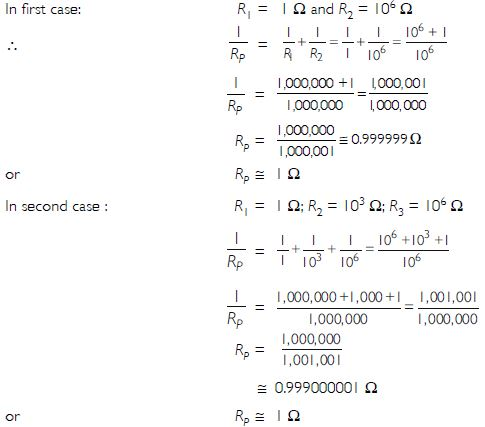##### Question 16:

An electric lamp of 100 $\mathrm{\Omega }$, a toaster of resistance 50 $\mathrm{\Omega }$ and a water filter of resistance 500 $\mathrm{\Omega }$ are connected in parallel to a 220 V source. What is the resistance of the electric iron connected to the same source that takes as much current as all the three appliances and what is the current through it?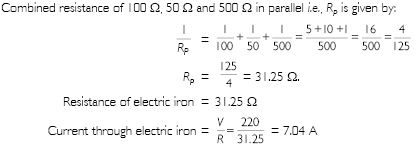##### Question 17:

What are the advantages of connecting electric devices in parallel with the battery instead of connecting them in series?

When connected in parallel, all the electric devices are having same potential. When connected in series, the potential is divided between different devices. Thus, the devices will not work properly when connected in series.

##### Question 18:

How can three resistors of resistance 2$\mathrm{\Omega }$, 3$\mathrm{\Omega }$ and 6$\mathrm{\Omega }$ be connected to give a total resistance of
(a) 4 $\mathrm{\Omega }$
(b) 1 $\mathrm{\Omega }$?

(a) When 3 W and 6 W are in parallel,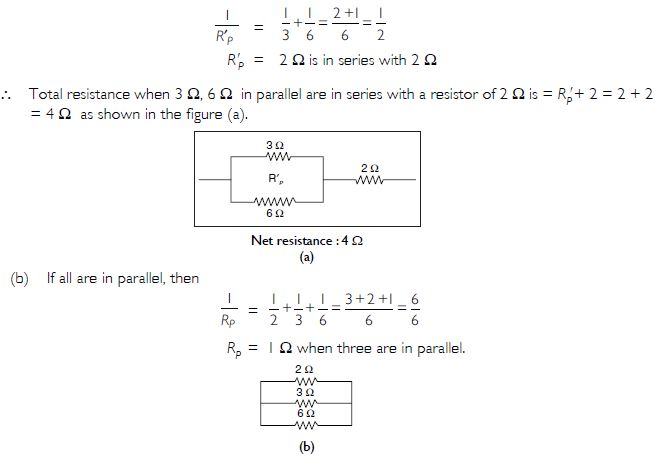##### Question 19:

What is the (a) highest; (b) lowest total resistance that can be secured by combination of four coils of resistances 4 $\mathrm{\Omega }$, 8 $\mathrm{\Omega }$, 12 $\mathrm{\Omega }$, 24 $\mathrm{\Omega }$?

Resistance is maximum when they are connected in series. Therefore, highest resistance = 4 + 8 + 12 + 24 = 48 W Resistance is lowest when they are connected in parallel.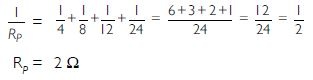##### Question 20:

Why does the cord of an electric heater not glow while the heating element does?

Heating effect = I2R, where I is the current flowing and R is the resistance. Current is same in both the cord and the heating element.
Resistance R of the cord is negligible since it is made of copper (which has very less resistivity), not heated much and hence, does not glow. On the other hand, nichrome has high resistance and gets heated up much more than copper and hence, glows.

##### Question 21:

Compute the heat generated while transferring 96,000 C of charge in one hour through a potential difference of 50 V.

Work done to transfer Q coulomb of charge through potential difference of V volt is = W = VQ joule
If Q = 96,000 C
then W = 50 × 96,000 = 48,00,000 J
W = 4.8 × 106 J
which is equal to the heat generated.

##### Question 22:

An electric iron of resistance 20 $\mathrm{\Omega }$ takes a current of 5 A. Calculate the heat developed in 30 s.

R = 20 $\mathrm{\Omega }$; I = 5 A; t = 30 s.
H = I2 Rt = (5)2 (20) (30)
H = 15,000 J = 15 kJ

##### Question 23:

What determines the rate at which the energy is delivered by a current?

Electric power determines the rate at which energy is delivered.
P = VI
If V = 1 volt; I = 1 ampere; then P = 1 watt
\ Power consumed by a device is 1 W if 1 A of current flows across a potential difference of 1 V.

##### Question 24:

An electric motor takes 5 A from a 220 V line. Determine the power of the motor and the energy consumed in 2 h.

Power = P = VI = 220 × 5 = 1,100 W
Energy consumed in 2 h (2 × 60 × 60 s) = E = Pt
E = (1,100) (2 × 60 × 60) = 7,920,000 J
E = 7.92 × 106 J

##### Question 25:

A piece of wire of resistance R is cut into five equal parts. These parts are then connected in parallel. If the equivalent resistance of this combination is R¢, then the ratio R/R is
(a) 1/25
(b) 1/5
(c) 5
(d) 25.

On cutting a wire of resistance R into five equal parts, the resistance of each part is R/5. The total resistance R¢ of 5 resistors R/5 each in parallel is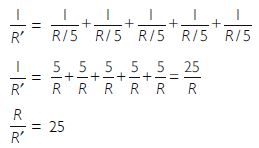##### Question 26:

Which of the following terms does not represent electrical power in the circuit? (a) I2R
(b) IR2
(c) VI
(d) V2/R

(b) because all other three represent power. Only IR2 is not power.

##### Question 27:

An electric bulb is rated 220 V and 100 W. When operated on 110 V, the power consumed will be (a) 100 W
(b) 75 W
(c) 50 W
(d) 25 W?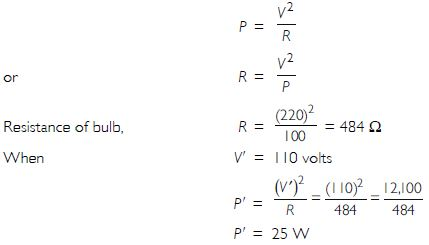##### Question 28:

Two conducting wires of the same material and of equal lengths and equal diameters are first connected in series and then in parallel in an electric circuit across the same potential difference. The ratio of heat produced in series and parallel combination would be
(a) 1 : 2
(b) 2 : 1
(c) 1: 4
(d) 4 : 1.

Since the wires are of the same material and are equal in lengths and diameters, therefore, resistance of both the wires is same. The resultant resistance of two resistances of two wires in series and parallel would be RS and RP respectively given by: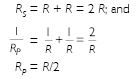When connected to a source V, then heat produced in series is HS and when in parallel is HP and is given by: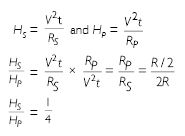i.e., HS : HP : : 1 : 4
i.e., (c) is correct.

##### Question 29:

How is a voltmeter connected in the circuit to measure potential difference between two points?

Voltmeter is always connected in parallel to the ends of the resistor across which the potential difference is to be measured.

##### Question 30:

A copper wire has diameter 0.5 mm and resistivity 1.6 × 10–8 Wm. What will be the length of this wire to make its resistance 10 $\mathrm{\Omega }$? How much does the resistance change if the diameter is doubled?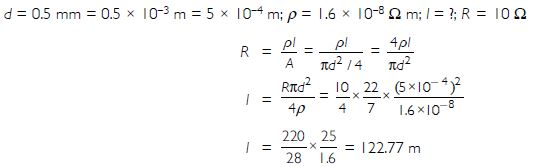If the diameter of wire is doubled, resistance will become 1/4th of the original.
(Since R a 1/A or R a 1/d2).
Decrease of resistance will be 10 – 10/4 = 7.5 W.

##### Question 31:

The values of the current I flowing in a given resistor for the corresponding values of potential difference V across the resistor are given below: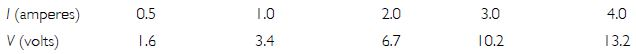Plot a graph between V and I and calculate the resistance of that resistor.
Plot a graph between V and I and calculate the resistance of that resistor.

##### Question 32:

When a 12 V battery is connected across an unknown resistor, there is a current of 2.5 mA in the circuit. Find the value of the resistance of resistor?

Here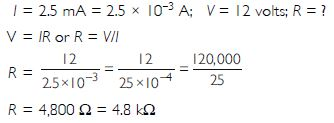##### Question 33:

A battery of 9 V is connected in series with resistors of 0.2 $\mathrm{\Omega }$, 0.3 $\mathrm{\Omega }$, 0.4 $\mathrm{\Omega }$, 0.5 $\mathrm{\Omega }$ and 12 $\mathrm{\Omega }$ respectively. How much current will flow through a 12 $\mathrm{\Omega }$ resistor?

RS = 0.2 + 0.3 + 0.4 + 0.5 + 12 = 13.4 $\mathrm{\Omega }$
or I = 0.6716 A
When in series, the current passing through each resistor is equal to the current in the circuit.
$\therefore$ Current through 12 W = 0.6716 A

##### Question 34:

How many 176 $\mathrm{\Omega }$ resistors in parallel are required to carry 5 A on a 220 V line?

In n resistors each of resistance R are connected in parallel, then net resistance RP is: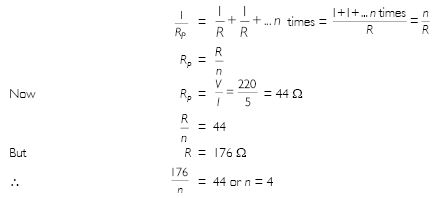4 resistors each of 176 W connected in parallel will result in net resistance of 44 W causing a current of 5 A to flow when connected to 220 V.

##### Question 35:

Show how will you connect three resistors, each of resistance 6 W, so that the combination has a resistance of (i) 9 $\mathrm{\Omega }$ (ii) 4 $\mathrm{\Omega }$?

(i) When two resistors each of 6 W are connected in parallel, they give resistance RP.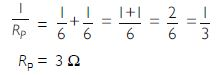When this combination is connected in series with third resistor of 6 W, it gives a total of 6 + 3 = 9W figure (a).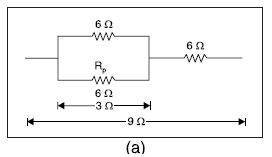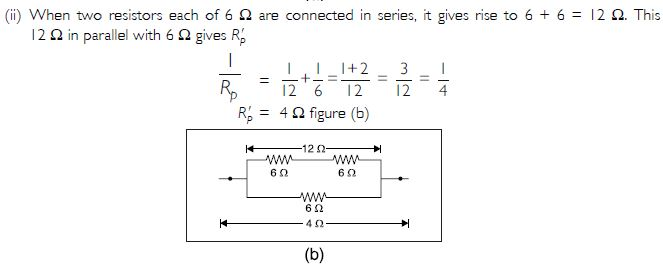##### Question 36:

Several electric bulbs designed to be used on a 220 V electric supply line are rated 10 W. How many lamps can be connected in parallel with each other across the two wires of 220 V line if the maximum allowable current is 5 A?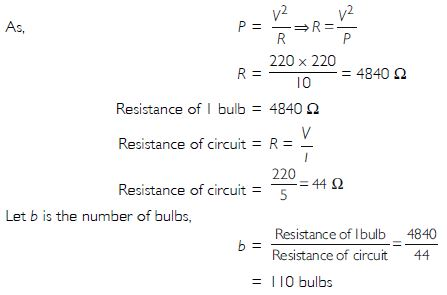##### Question 37:

A hot plate of an electric oven connected to a 220 V line has two resistance coils A and B, each of 24 $\mathrm{\Omega }$ resistance, which may be used separately, in series or in parallel. What are currents in three cases?

Each coil has a resistance of 24 W when used separately.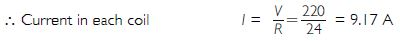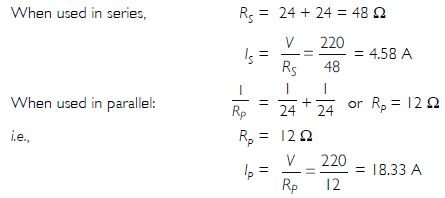##### Question 38:

Compare the power used in 2 W resistor in each of the following circuits (i) a 6 V battery in series with 1 $\mathrm{\Omega }$ and 2 $\mathrm{\Omega }$ resistors and (ii) a 4 V battery in parallel with 12 $\mathrm{\Omega }$ and 2$\mathrm{\Omega }$ resistors.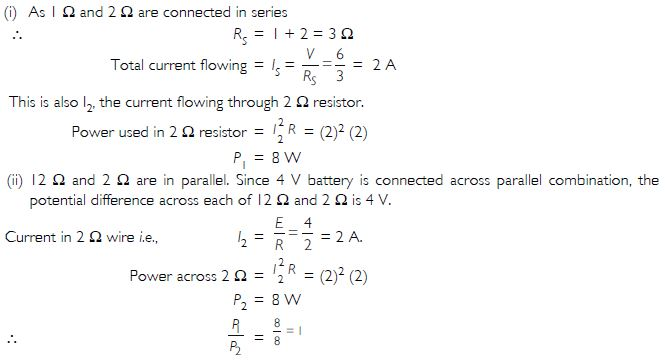##### Question 39:

Two lamps, one rated 100 $\mathrm{\Omega }$ at 220 V and other 60 $\mathrm{\Omega }$ at 220 V are connected in parallel to electric main supply. What current is drawn from the line if the supply voltage is 220 V?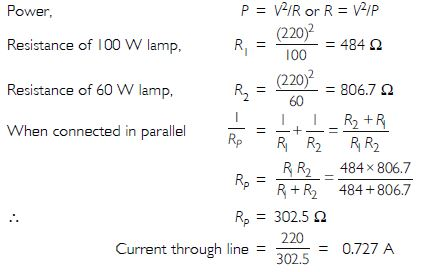##### Question 40:

Which uses more energy, a 250 $\mathrm{\Omega }$ TV set in 1 hour or a 1,200 $\mathrm{\Omega }$ toaster in 10 minutes?

Energy consumed, E1 = P1t1
= 250 W × 1 h
= 250 Js3 × 3,600 s
E1 = 900,000 J
E2 = P2t2
= 1,200 W × 10 min
E2 = 1,200 Js3 × 600 s
E2 = 720,000 J
$\therefore$ A 250 W TV set in 1h consumes more energy than a 1200 W toaster in 10 min.

##### Question 41:

An electric heater of resistance 8 $\mathrm{\Omega }$ draws 15 A from service mains for 2 hours. Calculate the rate at which heat is developed in the heater.

Rate at which heat is developed in the heater is its power.
P = I2R
= (15)2 (8) = 225 × 8
or P = 1,800 W or J s1
1,800 J of heat is developed per second.

##### Question 42:

Explain the following:
(a) Why is the tungsten used almost exclusively for filament of electric lamps?
(b) Why are the conductors of electric heating devices, such as bread toasters and electric irons, made of an alloy rather than a pure metal?
(c) Why is the series arrangement not used for domestic circuits?
(d) How does the resistance of a wire vary with its area of cross-section?
(e) Why are copper and aluminium usually employed for electricity transmission?